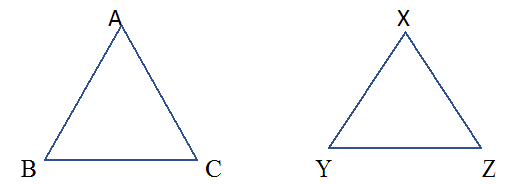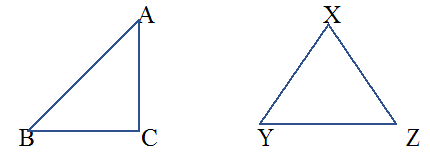# Ex.7.2 Q7 Congruence of Triangles - NCERT Maths Class 7

Go back to  'Ex.7.2'

## Question

In a squared sheet, draw two triangles of equal areas such that

(i) the triangles are congruent.

(ii) the triangles are not congruent.

What can you say about their perimeters?

Video Solution
Congruence Of Triangles
Ex 7.2 | Question 7

## Text Solution

Steps:

Draw two triangles of equal areas,

(i)Triangles $$ABC$$ and $$XYZ$$ have the same area and are congruent to each other. Also, perimeter of both the triangles will be equal.

Such that,  $$\overline{AB}\text{ }=\text{ }\overline{DE},\overline{BC}\text{ }=\text{ }\overline{EF}\;\rm{and}\;\overline{AC}\text{ }=\text{ }\overline{DF}$$
On adding, we get $$\overline{AB}+\overline{BC}+\overline{AC}=\overline{DE}+\overline{EF}+\overline{DF}$$
i.e, perimeter of both the triangles are equal.

(ii)Here, we have drawn two triangles $$ABC$$ and $$PQR$$ which are not congruent to each other.
Such that ,$$\overline{AB}\text{ }\ne \text{ }\overline{XY},\overline{BC}\text{ }\ne \text{ }\overline{YZ}\;\rm{and}\;\overline{AC}\text{ }\ne \text{ }\overline{XZ}$$
On adding, we get $$\overline{AB}+\overline{BC}+\overline{AC}=\overline{XY}+\overline{YZ}+\overline{XZ}$$
Also, the perimeter of both the triangles will not be the same.

Learn from the best math teachers and top your exams

• Live one on one classroom and doubt clearing
• Practice worksheets in and after class for conceptual clarity
• Personalized curriculum to keep up with school